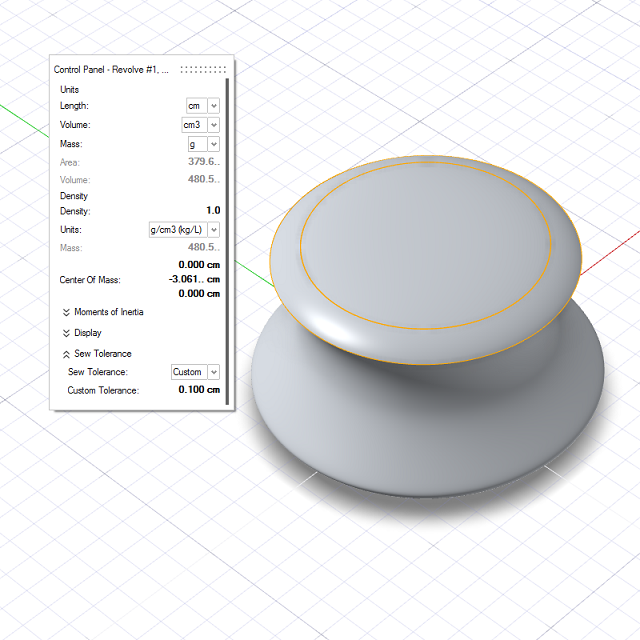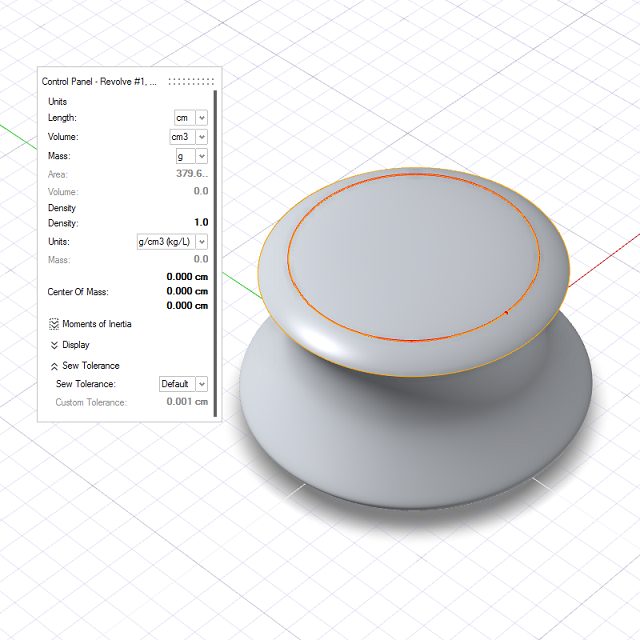# Area/Volume

Calculate the area, volume, mass, and moments of inertia of one or more objects. If an object isn't a closed solid, open edges are displayed in red.

## Calculate the Area and Volume

Calculate the area and volume of one or more objects.

If you select multiple surfaces that enclose a solid region, Inspire Studio automatically sews the surfaces together to calculate the volume of the solid. For example, if you select the faces of a cube, Inspire Studio automatically sews the faces together to calculate the volume of the cube.

1. On the ribbon, click the Analysis tab.
2. Click the Area/Volume icon.3. Click an object.
Note: To select multiple surfaces, box select them.
4. Choose from the following options:
Option Description
Units > Length Choose from the following units:
• mm
• cm (default)
• in
• ft
Units > Volume Choose from the following units:
• mm3
• cm3 (default)
• m3
• in3
• ft3
• ml
• cl
• l
Sew Tolerance Sew tolerance is used to sew adjacent edges. By default it is set to 0.001 cm. Change the method to custom to set a custom tolerance value. A higher value is more forgiving but less accurate.5. The area and volume are displayed.
6. Right-click and mouse through the check mark to exit, or double-right-click.

## Calculate the Mass and Moments of Inertia

Calculate the mass and moments of inertia of one or more objects.

1. On the ribbon, click the Analysis tab.
2. Click the Area/Volume icon.3. Click an object.
4. Choose from the following options:
Option Description
Units > Mass Choose from the following units:
• mg
• g (default)
• kg
• lb
Density Define the density, and choose from the following units:
• g/cm3 (kg/l)
• kg/m3
• lb/in3
• lb/ft3
Center of Mass Define the x, y, z coordinates of the center of mass.
Display > Center of mass Display the center of mass in the modeling window.
Display > Principal Axes Display the principal axes in the modeling window.
5. The following information is displayed:
Parameter Description
Mass The mass, based on the density and the units for mass.
Moments of Inertia
• Ixx Iyy Izz: The moments of inertia about the axes oriented as global x, y, z and passing through the center of mass.
• Iyz Ixz Ixy: The products of inertia about the axes oriented as global x, y, z and passing through the center of mass.
• Principal moments: The moments of inertia about the three principal axes.
• Principal axis X: The x, y, z coordinates of the first principal axis.
• Principal axis Y: The x, y, z coordinates of the second principal axis.
• Principal axis Z: The x, y, z coordinates of the third principal axis.
6. Right-click and mouse through the check mark to exit, or double-right-click.